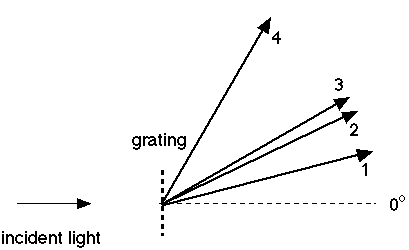# Grating Spectrometer

• Gee Wiz

## Homework Statement

White light illuminates a 2-cm wide diffraction grating at normal incidence. The dispersed light falls upon a viewing screen. The entire first-order and second-order patterns are observed. The four arrows in the figure below represent the first- and second-order red and blue bands. Let the wavelengths of red and blue light be 700 nm and 400 nm, respectively. Identify the color of each of the four bands by entering the wavelength of the light in the appropriate box.

Find the wavelength of the four bands.θ=1.22*λ/D

## The Attempt at a Solution

I figured(more like guessed) that bands 4 and 1 were 700nm and 400nm respectively, but I don't understand why. I have been trying to look through my notes and textbook to find some kind of equation or explanation, but so far to no avail. I am not really sure how to tackle this problem. I Kind of just looked at the bands realizing that 4 and 1 are first principle maximas and 3 and 2 are second principle maxs

Last edited:
Gee Wiz said:

## Homework Equations

θ=1.22*λ/D

This is not an equation that is applicable to a diffraction grating.

ya i just realized that is for circular slits